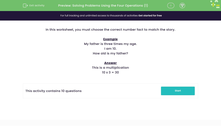# Solving Problems Using the Four Operations (1)

In this worksheet, students use their knowledge of addition, subtraction, multiplication and division to solve a range of problems.Key stage:  KS 2

Curriculum topic:   Number: Multiplication and Division

Curriculum subtopic:   Solve Multiplication/Division Problems

Popular topics:   Ratio worksheets

Difficulty level:#### Worksheet Overview

In this activity, we will be choosing facts to match a story.

First, we must decide what type of calculation is needed.

Then choose the right calculation.

Here is a division problem.

Ben has 36 toy cars and shares them into 3 piles.

The calculation needed is 36 ÷ 3

Let;s try an example question together.

Example

Tom's father is three times his age.

Tom is 10.

How old is Tom's father?

This is a multiplication problem.

We need to multiply Tom's age by 3.

10 x 3 = 30

Now it's your turn to try some questions like this. Good luck!

### What is EdPlace?

We're your National Curriculum aligned online education content provider helping each child succeed in English, maths and science from year 1 to GCSE. With an EdPlace account you’ll be able to track and measure progress, helping each child achieve their best. We build confidence and attainment by personalising each child’s learning at a level that suits them.

Get started